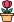엔지니어 게시판
LeetCode 솔루션 분류

# [1/14] 1061. Lexicographically Smallest Equivalent String

### 작성자 정보

•작성
• 작성일

• 119 조회
• 1 댓글
• 0 추천
• 0 비추천

### 본문

1061. Lexicographically Smallest Equivalent String

You are given two strings of the same length `s1` and `s2` and a string `baseStr`.

We say `s1[i]` and `s2[i]` are equivalent characters.

• For example, if `s1 = "abc"` and `s2 = "cde"`, then we have `'a' == 'c'``'b' == 'd'`, and `'c' == 'e'`.

Equivalent characters follow the usual rules of any equivalence relation:

• Reflexivity: `'a' == 'a'`.
• Symmetry: `'a' == 'b'` implies `'b' == 'a'`.
• Transitivity: `'a' == 'b'` and `'b' == 'c'` implies `'a' == 'c'`.

For example, given the equivalency information from `s1 = "abc"` and `s2 = "cde"``"acd"` and `"aab"` are equivalent strings of `baseStr = "eed"`, and `"aab"` is the lexicographically smallest equivalent string of `baseStr`.

Return the lexicographically smallest equivalent string of `baseStr` by using the equivalency information from `s1` and `s2`.

Example 1:

```Input: s1 = "parker", s2 = "morris", baseStr = "parser"
Output: "makkek"
Explanation: Based on the equivalency information in s1 and s2, we can group their characters as [m,p], [a,o], [k,r,s], [e,i].
The characters in each group are equivalent and sorted in lexicographical order.
```

Example 2:

```Input: s1 = "hello", s2 = "world", baseStr = "hold"
Output: "hdld"
Explanation: Based on the equivalency information in s1 and s2, we can group their characters as [h,w], [d,e,o], [l,r].
So only the second letter 'o' in baseStr is changed to 'd', the answer is "hdld".
```

Example 3:

```Input: s1 = "leetcode", s2 = "programs", baseStr = "sourcecode"
Explanation: We group the equivalent characters in s1 and s2 as [a,o,e,r,s,c], [l,p], [g,t] and [d,m], thus all letters in baseStr except 'u' and 'd' are transformed to 'a', the answer is "aauaaaaada".
```

Constraints:

• `1 <= s1.length, s2.length, baseStr <= 1000`
• `s1.length == s2.length`
• `s1``s2`, and `baseStr` consist of lowercase English letters.
Accepted
61.5K
Submissions
79.9K
<div
태그

댓글 1

## 학부유학생님의 댓글

• 익명
• 작성일
``````import collections
class Solution:
def smallestEquivalentString(self, s1: str, s2: str, baseStr: str) -> str:

lex_dic = collections.defaultdict(set)
LEN = len(s1)

for i in range(LEN):
res = []

memo = {}
def dfs(char, visited):
if char in memo: return memo[char]
res = char
for ch in lex_dic[char]:
if ch not in visited:
res = min(res, dfs(ch, visited))

return res

for char in baseStr:
visited = set()
min_char = dfs(char, visited)
res.append(min_char)
for c in visited: memo[c] = min_char
return "".join(res)``````
전체 349 / 1 페이지

• 등록일 02.01
• 등록일 02.01
• 등록일 02.01
• 등록일 02.01
• 등록일 02.01
• 등록일 02.01
• 등록일 02.01
• 등록일 02.01
• 등록일 02.01
• 등록일 02.01
• 등록일 02.01
• 등록일 02.01

• 등록일 01.31
• 등록일 01.31
• 등록일 01.31
• 등록일 01.27
• 등록일 01.27
• 등록일 01.23
• 등록일 01.23

### Stats

• 현재 접속자 50 명
• 오늘 방문자 123 명
• 어제 방문자 1,716 명
• 최대 방문자 2,853 명
• 전체 회원수 535 명